Full Text:<1978>

CLC number: TU433

On-line Access: 2013-06-03

Revision Accepted: 2013-04-10

Crosschecked: 2013-05-16

Cited: 2

Clicked: 4234

Citations:  Bibtex RefMan EndNote GB/T7714

 Journal of Zhejiang University SCIENCE A 2013 Vol.14 No.6 P.435-446 http://doi.org/10.1631/jzus.A1200343

One-dimensional nonlinear consolidation of soft clay with the non-Darcian flow*

 Author(s):  Chuan-xun Li1,2, Kang-he Xie2 Affiliation(s):  1. Faculty of Civil Engineering and Mechanics, Jiangsu University, Zhenjiang 212013, China; more Corresponding email(s):   lichuanxun@yeah.net Key Words:  Non-Darcian flow, Nonlinear consolidation, Time-dependent loading, Uniform distribution of self-weight stress, Linear increment of self-weight stress Share this article to： More <<< Previous Article|Next Article >>>

Chuan-xun Li, Kang-he Xie. One-dimensional nonlinear consolidation of soft clay with the non-Darcian flow[J]. Journal of Zhejiang University Science A, 2013, 14(6): 435-446.

@article{title="One-dimensional nonlinear consolidation of soft clay with the non-Darcian flow",
author="Chuan-xun Li, Kang-he Xie",
journal="Journal of Zhejiang University Science A",
volume="14",
number="6",
pages="435-446",
year="2013",
publisher="Zhejiang University Press & Springer",
doi="10.1631/jzus.A1200343"
}

%0 Journal Article
%T One-dimensional nonlinear consolidation of soft clay with the non-Darcian flow
%A Chuan-xun Li
%A Kang-he Xie
%J Journal of Zhejiang University SCIENCE A
%V 14
%N 6
%P 435-446
%@ 1673-565X
%D 2013
%I Zhejiang University Press & Springer
%DOI 10.1631/jzus.A1200343

TY - JOUR
T1 - One-dimensional nonlinear consolidation of soft clay with the non-Darcian flow
A1 - Chuan-xun Li
A1 - Kang-he Xie
J0 - Journal of Zhejiang University Science A
VL - 14
IS - 6
SP - 435
EP - 446
%@ 1673-565X
Y1 - 2013
PB - Zhejiang University Press & Springer
ER -
DOI - 10.1631/jzus.A1200343

Abstract:
Based on the non-Darcian flow law described by exponent m and threshold gradient i 1 under a low hydraulic gradient and the classical nonlinear relationships e-lgσ′ and e-lgk v (Mesri and Rokhsar, 1974), the governing equation of 1D nonlinear consolidation was modified by considering both uniform distribution of self-weight stress and linear increment of self-weight stress. The numerical solutions for the governing equation were derived by the finite difference method (FDM). Moreover, the solutions were verified by comparing the numerical results with those by analytical method under a specific case. Finally, consolidation behavior under different parameters was investigated, and the results show that the rate of 1D nonlinear consolidation will slow down when the non-Darcian flow law is considered. The consolidation rate with linear increment of self-weight stress is faster than that with uniform distribution one. Compared to Darcy’s flow law, the influence of parameters describing non-linearity of soft soil on consolidation behavior with non-Darcian flow has no significant change.

1.  Introduction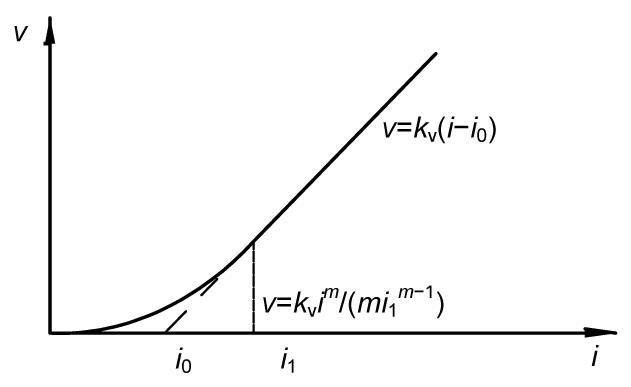Fig.1
Relationship between flow velocity, v, and hydraulic gradient, i

Since the non-Darcian flow has been observed in some permeability tests of fine-grained soils, and the non-Darcian flow law proposed by Hansbo () has been recognized by many researchers, it has a theoretical significance in associating the influences of this non-Darcian flow law on consolidation behavior. Dubin and Moulin () replaced the exponential relation with a linear relation at the case of i<i l, and firstly investigated the problem of 1D consolidation with this non-Darcian flow. Hansbo (; ) analyzed 1D consolidation and uncoupled consolidation with vertical drains by adopting this non-Darcian flow, and observed a better agreement to the field settlement observations. Moreover, based on this non-Darcian flow, Ing and Nie () analyzed the consolidation of sand-drained ground with the radial and vertical drainages, and investigated the influence of non-Darcian flow on the consolidation behavior. Xie et al. () completely neglected the water flow under the case that hydraulic gradient was less than threshold hydraulic gradient, and provided an approximate solution for the problem of 1D consolidation with threshold gradient. Li et al. (; ) performed a comprehensive analysis of 1D consolidation, considering this non-Darcian flow, along with the change of vertical total stress depending on both depth and time.

All these previous studies with this non-Darcian flow, however, neglected the non-linear compressibility and permeability of soft soil during the process of consolidation. In practice, the non-linear compressibility and permeability of soil in the process of consolidation has been realized for 50 years (Davis and Raymond, ), and many results on non-linear consolidation with Darcy’s flow law have been obtained. However, no study is made to consider non-linear compressibility and permeability of soft soil along with non-Darcian flow simultaneously. Since both non-Darcian flow in soft soil and non-linear compressibility and permeability of soil can be observed during the process of consolidation, it has a theoretical and practical significance in acquainting the influence of this non-Darcian flow and nonlinearity of clay on consolidation behavior.

2.  Theoretical formulation of 1D nonlinear consolidation with non-Darician flow

2.1.  Presentation and basic assumptions

In this study, the clay layer, with a thickness of H, is assumed to be fully saturated. The top surface of clay layer is pervious, and the bottom is impervious. Fig. 2 shows the time-dependent loading used in this study, which increases with time up to the final value and then remains constant. The initial value, the final value, and the construction time are denoted as q 0, q u, and t c, respectively. A particular time-dependent loading, ramp loading, also is shown as a dashed line in Fig. 2. To investigate the consolidation behavior with consideration of non-Darcian flow law and nonlinearity of soft soil, the following assumptions are made:

1. The soil grains and pore water in soil are incompressible.

2. The soil only has a small deformation.

3. The void ratio of soil changes with effective stress is given as , where e is the void ratio, σ′ is the effective stress, σ 1′ is an known effective stress, e 1 is an known void ratio corresponding to σ 1′, and cc is the compressibility index.

4. The water flow in soil follows Eq. (1), and the parameters m and i 1 maintain constant during consolidation. As noted by Mesri and Rokhsar (), a linear e-lgk v relation may represent permeability behavior of most natural soft clays with small strains. Therefore, the coefficient of permeability may decrease as per: , where k v1 is the coefficient of permeability corresponding to the void ratio e 1, and ck is the permeability index.

5. The initial effective stress (self-weight stress) is assumed to remain constant or increase with depth linearly in this study. If the self-weight stress σ 0′ is assumed to remain constant, a known effective stress σ 1′ is equal to σ 0′, that is σ 1′=σ 0′. If the self-weight stress σ 0′ is assumed to increase with depth linearly, σ 0′=γz, where γ′ is the effective unit weight, and z is the ordinate. Moreover, the average value of self-weight stress within the whole soil layer is denoted as σ 1′, i.e., σ 1′=0.5γH, where H is the thickness of soil layer. These two kinds of distribution of self-weight stress may be shown as Fig. 3.

2.2.  Development of governing equations

From Eqs. (2) and (3), the coefficient of volume compressibility m v and coefficient of permeability k v can be obtained: , , where m v1 is the coefficient of volume compressibility corresponding to the known effective stress σ 1′, which can be expressed as .

Since the soil only occur elastic small deformation, the general continuity equation for 1D consolidation can be expressed as , where e 0 is the initial void ratio of the soil, and it is corresponding to the self-weight stress σ 0′ in the linear relation of e-lgσ′.

With Eqs. (1), (4), (5), and (6), the governing equation with the non-Darcian flow and non-linear compressibility and permeability of soil can be obtained: where γ w is the unit weight of water, u(z, t) is the excess pore water pressure depended on z and t, and c v1=k v1/(γ w m v1).

According to the principle of effective stress, , Eq. (7) can be further expressed as

With pervious top and impervious bottom, the vertical boundary conditions can be written as

The initial condition is .

Eq. (8) is the governing equation of 1D consolidation considering non-Darcian flow and non-linearity of compressibility and permeability. This equation is a second-order non-linear partial differential equation, and it can be rewritten as , where indicates the change of coefficient of permeability with consideration of non-Darcian flow and non-linear permeability. This coefficient can be determined by the pore water pressure and the hydraulic gradient to be: m v(u) indicates the change of coefficient of volume compressibility with consideration of non-linear compressibility of soil. It can be determined by the excess pore water pressure to be: .

As shown in Eq. (11), Eq. (8) may be regarded as a quasi-linear diffusion equation. Because of the complexity of this second-order quasi-linear diffusion equation, the analytic solutions for Eq. (8) cannot easily be obtained. Therefore, the finite difference method is adopted to develop the numerical solutions for Eq. (8) in this study.

3.  Derivation of numerical solutions

3.1.  Governing equations in terms of dimensionless variables

For convenience of derivations, the following dimensionless variables are defined as

In terms of these dimensionless variables, the governing Eq. (8) can be rewritten as

Eqs. (9) and (10) can be rewritten as .

3.2.  Numerical solution for pore water pressure

To obtain numerical solutions for Eq. (15) by the FDM, the spatial domain 0≤Z≤1 is firstly divided into n thin layers with equal length ΔZ, and the jth spatial nodal point is denoted as .

Z 0 and Zn indicate the top and bottom surfaces of the soil layer, respectively. Meanwhile, the time axis is divided by a series of time increment, and the time increment is denoted as ΔT v. Therefore, the kth time nodal point can be written as .

In terms of dimensionless variable, the average value of self-weight stress in the jth thin layer may be denoted as Sj . With the assumption 5, if the self-weight stress is supposed to maintain constant, Sj =1; if the self-weight stress is supposed to change with depth linearly, Sj can be expressed as .

As is well known, difference scheme greatly influences the accuracy and stability of the FDM. The Crank-Nicolson difference scheme is usually convergent, but the corresponding expression is complicated. The expression for explicit difference scheme is simple, but the convergence of explicit difference scheme highly depends on the value of time step ΔT v. Since Eq. (15) essentially is a quasi-linear diffusion equation, linearization technique may be employed to obtain difference solutions for quasi-linear diffusion equation. For example, considering variable coefficients as constants for a given tiny time increment, the exact solution can be obtained by iteration. Therefore, an implicit difference scheme of quasi-linear diffusion equation can be adopted to obtain numerical solutions, due to its relative simplicity and good convergence. In terms of the implicit difference scheme of the quasi-linear diffusion equation, the following difference equation can be obtained: , where Uj k is the dimensionless variable of excess pore water pressure at Z=jΔZ and T v=T vk , Qk is the dimensionless variable of time-dependent loading at T v=T vk , λT v/(ΔZ)2, j=1, 2, …, n, k=0, 1,…, and is decided by where , is defined as and is defined as

Difference Eq. (21) is a series of non-linear equations of unknown variables Uj k +1, and thus an iteration method must be adopted to obtain exact solutions for Uj k +1. Uj k can be adopted as the initial iterative value to obtain the values of , and . In addition, the iteration would be governed by either iterative frequency or calculation accuracy, and the smaller the value of ΔT v, the less the iterative frequency.

In terms of discrete nodal points, the boundary and initial conditions, specified by Eqs. (16) and (17), respectively, can be written as follows: , .

Eqs. (21), (24) and (25) compose a closed system of equations, and the exact solutions for unknown variables Uj k +1 can be obtained by the method of iteration.

3.3.  Numerical solution for average degree of consolidation

The average degree of consolidation in terms of pore water pressure is the ratio of the average effective stress at any time to the final average effective stress, and that at T vk follows:

The average degree of consolidation in terms of deformation is the ratio of the settlement at any time to the final settlement, and that at T vk can be expressed as where εzt is the soil strain of depth z at time t; εzf is the final soil strain of the depth z.

From Eqs. (26) and (27), it can be seen that the average degree of consolidation defined by the water pressure is different from that defined by the deformation; that is, the dissipation rate of pore water pressure is different from deformation rate with consideration of non-Darcian flow and non-linearity of compressibility and permeability.

4.  Verification of the numerical solutions

If the exponent m is equal to 1, Eq. (1) degenerates into Darcy’s flow law. In addition, the coefficient of consolidation is assumed to remain constant during the process of consolidation; that is, the ratio of compressibility index cc to permeability index ck is equal to 1. Under these assumptions, Xie et al. () obtained an analytical solution for Eq. (8) with consideration of ramp loading and uniform distribution of self-weight stress. To verify the accuracy of the aforementioned finite difference scheme, a comparison is made between the results by the present numerical method and the analytical method based on m=1, S=1, and c=1 (Table 1).

Table 1

Comparison of the results by FDM with that by analytical method (n=100, ΔT v=1×10−6)
 T v c=1, b=4, m=1, T vc =0.1, S=1 c=1, b=8, m=1, T vc =0.05, S=1 U p U s U p U s FDM (%) Analytical method (%) Error (%) FDM (%) Analytical method (%) Error (%) FDM (%) Analytical method (%) Error (%) FDM (%) Analytical method (%) Error (%) 0.002 0.0674 0.0732 0.0058 0.1435 0.1483 0.0048 0.1313 0.1325 0.0012 0.413 0.4112 −0.0018 0.005 0.262 0.268 0.006 0.5457 0.5501 0.0044 0.4953 0.4965 0.0012 1.433 1.4297 −0.0033 0.01 0.7271 0.7337 0.0066 1.4633 1.4673 0.004 1.3245 1.3262 0.0017 3.4376 3.4333 −0.0043 0.03 3.5656 3.5763 0.0107 6.4088 6.4144 0.0056 6.0064 6.0132 0.0068 11.8625 11.8591 −0.0034 0.05 7.3414 7.356 0.0146 12.0867 12.0925 0.0058 11.9204 11.9324 0.012 19.8463 19.8419 −0.0044 0.08 14.1041 14.1255 0.0214 20.9163 20.9227 0.0064 17.4128 17.4164 0.0036 28.1542 28.1489 −0.0053 0.1 19.1576 19.1839 0.0263 26.8097 26.8165 0.0068 20.0994 20.1036 0.0042 32.4073 32.4031 −0.0042 0.2 33.2753 33.2945 0.0192 45.0848 45.0967 0.0119 30.461 30.4771 0.0161 48.2026 48.2141 0.0115 0.4 52.8413 52.8612 0.0199 66.6586 66.6678 0.0092 48.2299 48.2483 0.0184 68.4727 68.4822 0.0095 0.56 65.4577 65.4781 0.0204 77.5347 77.5422 0.0075 60.9367 60.9562 0.0195 78.756 78.7637 0.0077 0.8 79.2945 79.3142 0.0197 87.5729 87.5782 0.0053 75.861 75.8801 0.0191 88.2484 88.2538 0.0054 1 86.8446 86.8631 0.0185 92.4127 92.4165 0.0038 84.4192 84.4370 0.0178 92.8251 92.8289 0.0038

Table 1 reveals a good agreement between the FDM and the analytical method. Furthermore, the maximal error is less than 0.03%. It confirms that the FDM is reliable in calculating 1D non-linear consolidation with Darcy’s flow law. The main difference between Darcy’s flow law and non-Darcian flow law (assumed here) lies in the values of and . Under the case of Darcy’s flow law, Eqs. (22) and (23) may be converted into: , .

However, for the case of non-Darcian flow law, the values of and may be decided by Eqs. (22) and (23). Based on the reliability of the FDM in calculating the 1D non-linear consolidation with Darcy’s flow law, if the expressions for and in the case of non-Darcian flow law can be ensured to be correct in the finite difference programming, and the implicit difference scheme of quasi-linear diffusion equation is convergent, while the method of iteration is adopted during the process of calculation, the results with non-Darcian flow by the finite difference method should also be reliable.

5.  Analysis of consolidation behavior

Hansbo (; ; ) reported that the value of m was equal to 1.5 for the typical Swedish clays. In this study, the value of m is assumed to range from 1 to 2. For the value of i 1, Hansbo () stated that i 1 ranged from 4 to 10, and Dubin and Moulin () reported that i 1 was between 8 and 35, while Tavenas et al. (1983) indicated that i 1 may be less than 0.2. To assess the impact of i l on consolidation behavior, in this study, i l is assumed to vary within 0.5–40. Mesri and Rokhsar () suggested that the ratio of cc to ck for most soft soil usually ranged from 0.5 to 2. To investigate the influence of non-linear compressibility and permeability on consolidation behavior, the value of c was selected in the range of 0.5–2 in this study.

5.1.  Influence of m and i1 on consolidation behavior

Li et al. () studied the influence of non-Darcian flow on 1D consolidation without consideration of non-linearity of compressibility and permeability, which indicated that the dissipation rate of pore water pressure would decrease with the increasing value of m or i 1. From Figs. 4 and 5, the influence of m and i 1 on the dissipation of excess pore water pressure can be observed considering non-linearity of soft soil. In the case that the self-weight stress changes linearly with depth, the dissipation of excess pore water pressure in soil layer may be retarded with consideration of non-Darcian flow. In addition, the larger the values of m or i 1, the larger the residual pore water pressure. In other words, this retarded dissipation of pore water pressure would become more evident with an increase of m or i 1. This consolidation behavior can be explained from Fig. 1 and Eq. (1) that the velocity of seepage may slow down with an increase of i 1 or m. As a result, the dissipation rate of excess pore water pressure may be retarded.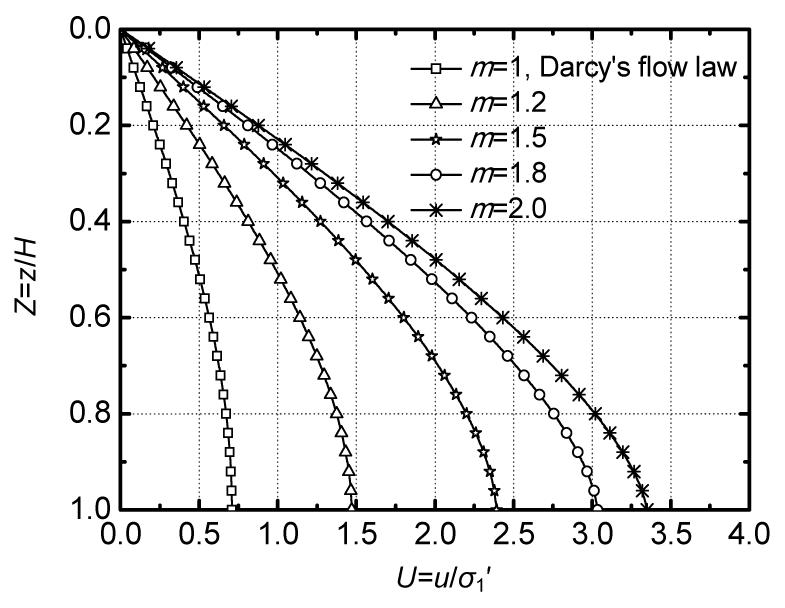Fig.4
Influence of m on the dissipation of excess pore water pressure
c c=0.65, c=0.5, e 1=1.0, i 1=4, σ 1′=0.5γH, q u=2γH, T vc =0.01, and T v=0.5; linear distribution of self-weight stressFig.5
Influence of i 1 on the dissipation of excess pore water pressure
cc =0.65, c=0.5, e 1=1.0, m=1.2, σ 1′=0.5γH, q u=1.5γH, T vc =0.01, and T v=0.6; linear distribution of self-weight stress

As stated above, since the dissipation rate of pore water pressure will slow down with consideration of non-Darcian flow, the consolidation deformation rate should also be retarded.

As show in Figs. 6 and 7, the consolidation rate would decrease with the increasing values of m or i 1. Moreover, if m=1.2 and i 1=4, the maximum difference in the average degree of consolidation indicated from Fig. 6a between Darcy’s flow law and non-Darcian flow law is 7.0%. If m=1.2 and i 1=5, as shown in Fig. 7, the corresponding maximum difference is 9.2%. Therefore, if m and i 1 are less than a certain value, the influence of non-Darcian flow on consolidation rate under a certain ratio of external loading to the average value of self-weight stress may be neglected. That is, non-Darcian flow law can be replaced by Darcy’s flow law in calculating the consolidation rate in some cases, even if the water flow in fine-grained soil may deviate from Darcy’s flow law. For instance, if i 1 is small enough (Fig. 6b, i 1=0.5), consolidation curves with different m are almost coincident each other, and Darcy’s flow law can be adopted to obtain the consolidation deformation.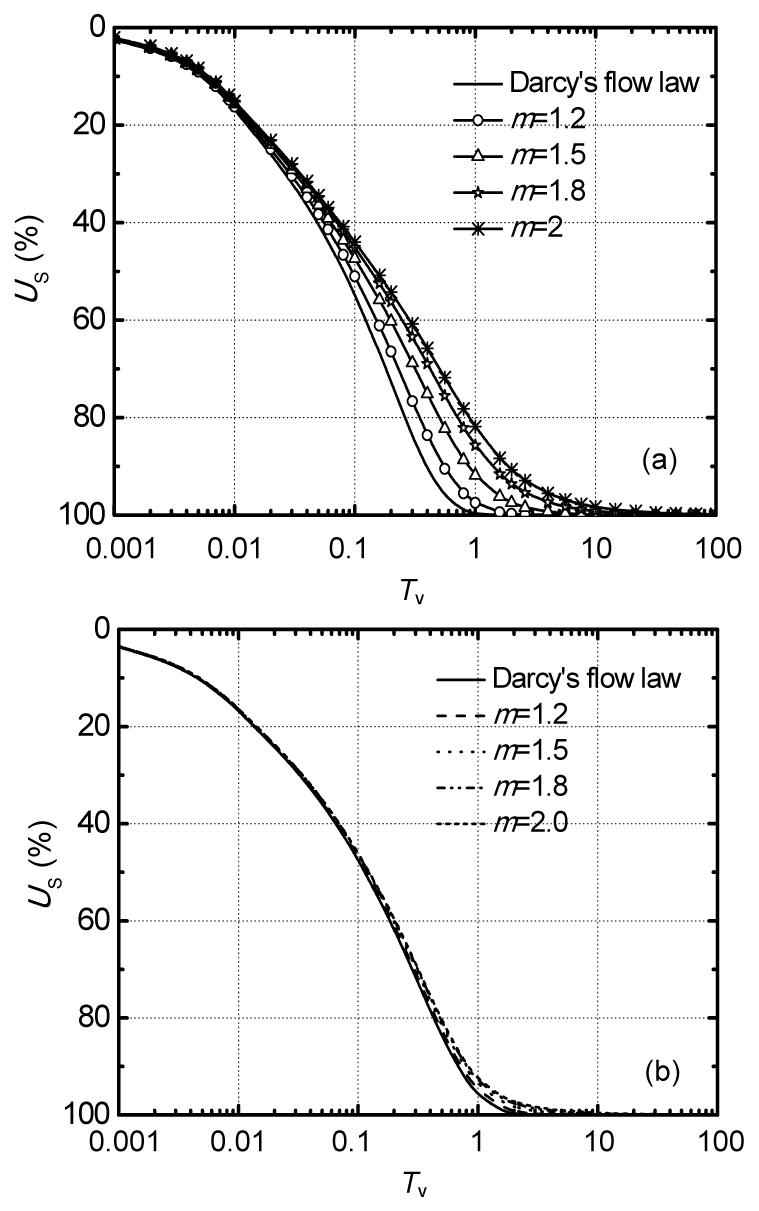Fig.6
Influence of m on the rate of consolidation deformation
(a) i 1=4, c=0.5; (b) i 1=0.5, c=1.0 cc =0.65, e 1=1.0, σ 1′=0.5γH, q u=2γH, and T vc =0.01; linear distribution of self-weight stress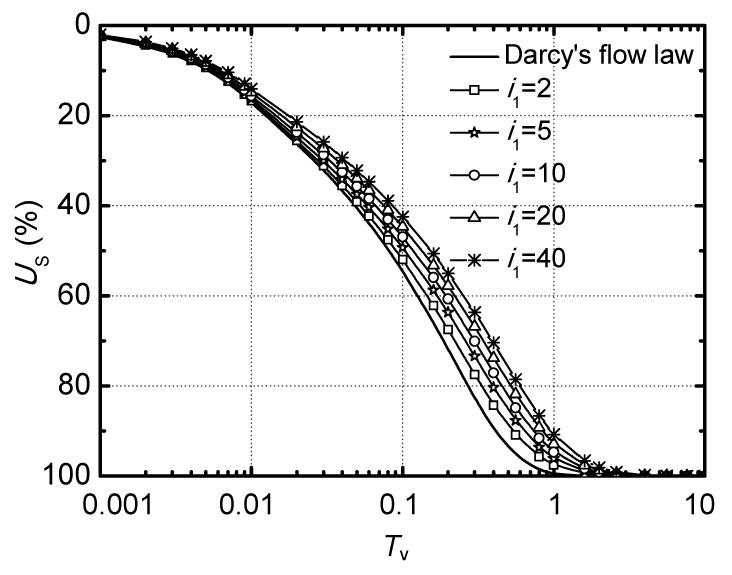Fig.7
Influence of i 1 on the rate of consolidation deformation
m=1.2, cc =0.65, c=0.5, σ 1′=0.5γH, q u=1.5γH, e 1=1.0, and T vc =0.01; linear distribution of self-weight stress

5.2.  Influence of external loading and thickness of soil layer on consolidation behavior

The influence of the ratio of q u to σ 1′ on the consolidation deformation rate under different cases of self-weight stress can be observed from Fig. 8. If self-weight stress remains constant with depth, as shown in Fig. 8a, the larger the ratio of q u to σ 1′, the faster the consolidation rate under c=0.5; but the consolidation rate decreases with the increasing ratio of q u to σ 1′ under c=2. In addition, b=1 indicates that non-linearity is not considered during the process of consolidation. The same consolidation behavior also can be observed from Fig. 8b with linear distribution of self-weight stress. When c=0.5, the larger the ratio of q u to σ 1′, the faster the deformation rate. When c=1.5, however, the deformation rate decreases with the increasing ratio of q u to σ 1′. This consolidation behavior can be explained from Eq. (15), if c<1, the value of (S+QU)c would increase with an increase in the ratio of q u to σ 1′, and the consolidation rates naturally step up. However, if c>1, the value of (S+QU)c would decrease with an increase in the ratio of q u to σ 1′, and the consolidation rates naturally slow down.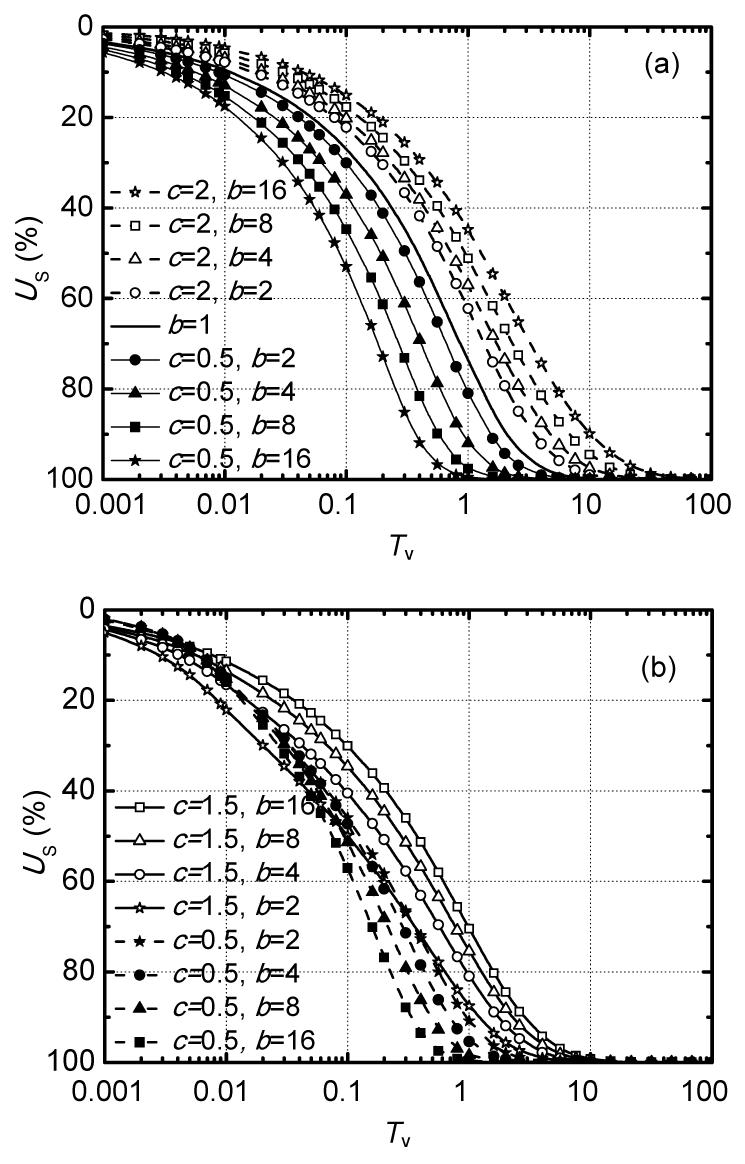Fig.8
Influence of the ratio of q u to σ 1′ on the rate of consolidation deformation
(a) Uniform distribution of self-weight stress, m=1.2, i 1=10, σ 1′=0.5γH, and T vc =0; (b) Linear distribution of self-weight stress, m=1.2, i 1=10, σ 1′=0.5γH, T vc =0.01, cc =0.65, and e 1=1.0

As mentioned above, the values of m and i 1 have a great influence on the difference of consolidation rate between Darcy’s flow law and non-Darcian flow law. In fact, the ratio of q u to σ 1′ also greatly influences this difference. As shown in Fig. 9, the difference of consolidation rate between m=1 and m=1.5 with b=17 is much less than that with b=2. As is well known, the average hydraulic gradient in soft soil may increase with an increase in the ratio of q u to σ 1′, then v-i curves have more opportunity to obey a linear relationship. As a result, the difference of consolidation rate between Darcy’s flow law and non-Darcian flow law can gradually reduce with an increase of the ratio of q u to σ 1′. That is, with an increase of q u or a reduction of H, the ratio of q u to σ 1′ should increase, and then the difference of the consolidation rate between Darcy’s flow law and non-Darcian flow would gradually lessen. Therefore, if a large load was applied to the surface of a thin soil layer, the non-Darcian flow may not be considered for its complexity, even if the water flow in soil under low gradient may obey the non-Darcian flow law.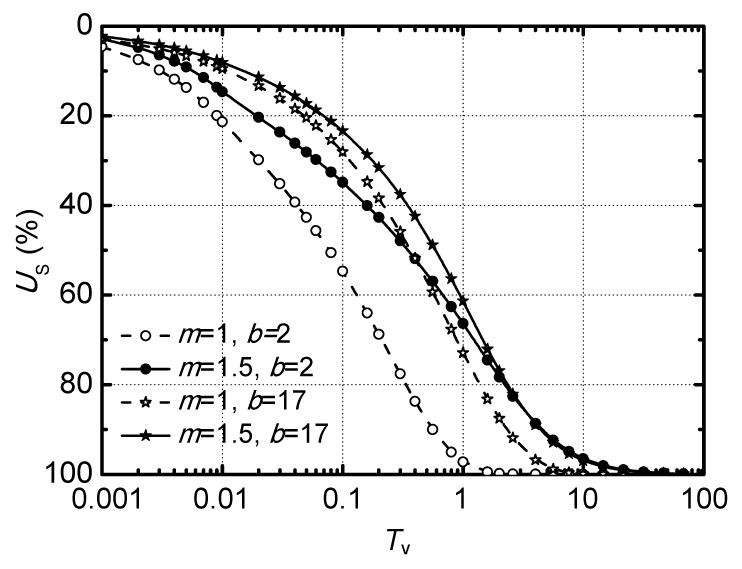Fig.9
Influence of the ratio of q u to σ 1′ on the difference of consolidation rate between Darcy’s flow and non-Darcian flow
σ 1′=0.5γH, T vc =0.01, i 1=10, c=1.5, cc =0.65, and e 1=1.0; linear distribution of self-weight stress

5.3.  Influence of cc and ck on consolidation behavior

From Fig. 10, the influence of c, the ratio of cc to ck , on the average degree of consolidation in terms of deformation can be observed. If the self-weight stress is supposed to be uniformly distributed with depth (Fig. 10a), the average degree of consolidation in terms of deformation decreases with an increase in the value of c. If the self-weight stress is supposed to increase linearly with depth (Fig. 10b), however, the consolidation rate increases with the increasing value of c at the early stage, and decreases with the increasing value of c at the final stage. This consolidation behavior lies in the fact that, at the early stage of consolidation, the value of (S+QU) with linear distribution of self-weight stress may be less than 1, and the value of (S+QU)c may increase with an increase of c. According to Eq. (15), the consolidation rate may accelerate with the increasing value of c at the early stage. However, if the self-weight stress remains constant or the excess pore water pressure with linear increase of self-weight stress has partially dissipated, the value of (S+QU) may be more than 1, and the value of (S+QU)c may decrease with an increase of c. As a result, the consolidation rate would slow down with an increase of c.Fig.10
Influence of the ratio of cc to ck on the rate of consolidation deformation
(a) Uniform distribution of self-weight stress; m=1.2, i 1=10, σ 1′=0.5γH, q u=1.5γH, and T vc =0.01; (b) Linear distribution of self-weight stress; m=1.2, i 1=10, σ 1′=0.5γH, q u=2.0γH, T vc =0.05, cc =0.75, and e 1=1.0

For the case of uniform distribution of self-weight stress, the average degree of consolidation, both in terms of stress and deformation, depends on the ratio of cc to ck , but is irrelative with the specific values of cc to ck . If the self-weight stress changes linearly with depth (Fig. 11), however, the rates of consolidation deformation with the same c and different c c are different, while the dissipation rates of pore water pressure with the same c and different cc are equal. In addition, the deformation rate increases with the decreasing value of cc . This consolidation behavior can be explained by Eq. (27). If the self-weight stress remains constant with depth, that is, Sj =1, only the value of c has influence on the dissipation of excess pore water pressure, and U p, U s may be independent of the specific value of cc . Otherwise, the value of cc may influence the final settlement of soil layer, and both c and cc have a great influence on the deformation rate.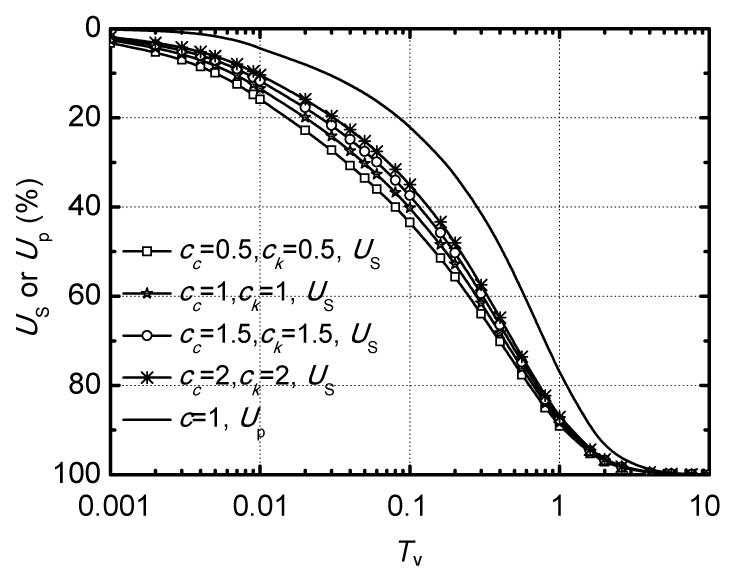Fig.11
Influence of cc on consolidation rate with the same ratio of cc to ck
Linear distribution of self-weight stress; m=1.2, i 1=10, σ 1′=0.5γH, q u=2.0γH, T vc =0.01, and e 1=1.0

5.4.  Influence of distribution of self-weight stress on consolidation behavior

To analyze the influence of distribution types of self-weight stress, both a uniform distribution and a linear increase of self-weight stress are incorporated in this study, and the difference between them is discussed. Fig. 12 shows that the consolidation rate with a linear distribution of self-weight stress is faster than that with a uniform distribution one (the value is equal to the average self-weight stress in the whole soil layer). In addition, it should be noted that the difference of consolidation rate between different distribution types of self-weight stress may gradually lessen with an increase in the ratio of q u to σ 1′. The appearances of consolidation behavior are due to the fact that the value of (S+QU)c with a linear distribution of self-weight stress is larger than that with a uniform distribution one. Furthermore, if the value of the ratio of q u to σ 1′ is small, the distribution types of self-weight stress may have a great influence on the value of (S+QU)c . Therefore, if external loading applied to the surface of soil layer is large enough, or the thickness of soil layer is thin enough (as shown in Fig. 12, b=17), the difference of consolidation rate between linear distribution and uniform distribution of self-weight stress should be fractional and acceptable.Fig.12
Influence of distribution types of self-weight stress on the rate of consolidation deformation
m=1.5, i 1=5, σ 1′=0.5γH, T vc =0.05, e 1=1.0, cc =0.75, and c=0.5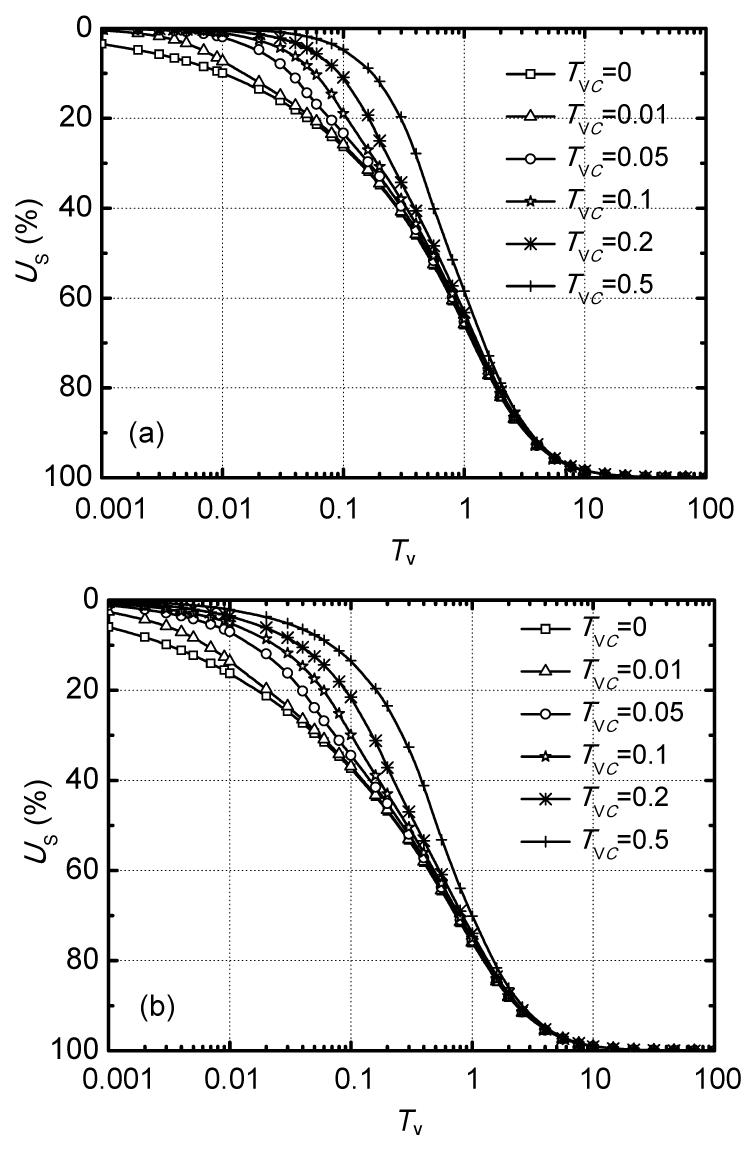Fig.13
(a) Uniform distribution of self-weight stress; m=1.5, i 1=10, c=1.0, σ 1′=0.5γH, and q u=2.0γH; (b) Linear distribution of self-weight stress. m=1.5, i 1=10, σ 1′=0.5γH, q u=2γH, e 1=1.0, c c=0.7, and c=1.0

6.  Conclusions

In this study, a 1D non-linear consolidation theory with non-Darcian flow law was developed, and numerical solutions were obtained by the FDM. The 1D non-linear consolidation behavior with non-Darcian flow law under different parameters was analyzed, and the following conclusions can be obtained.

1. The rate of 1D non-linear consolidation will slow down when considering the non-Darcian flow described by the exponent m and threshold hydraulic gradient i 1. In addition, this retardation of consolidation rate would intensify with an increase in the value of m or i 1.

2. If m or i 1 keep constant, the retardation of consolidation rate will reduce with an increase in the ratio of the final loading to average self-weight stress. Therefore, if a large load was applied to the surface of a thin soil layer, the non-Darcian flow for fine grained soil under low gradient can be replaced by Darcy’s flow law in calculating consolidation deformation.

3. When non-Darcian flow is considered, the influence of parameters describing non-linearity of soft soil on consolidation behavior has no significant change compared with that under Darcy’s flow law.

4. The consolidation rate with a linear increase of self-weight stress is faster than that with a uniform one. Moreover, the difference of consolidation rate between them will reduce with the increasing ratio of the final loading to average self-weight stress.

5. For both uniform distribution and linear increment of self-weight stress, the faster the loading rate, the faster the consolidation rate.

* Project supported by the National Natural Science Foundation of China (No. 51109092), the National Science Foundation for Post-doctoral Scientists of China (No. 2013M530237), and the Jiangsu University Foundation for Advanced Talents (No. 12JDG098), China

ReferencesDavis, E.H., Raymond, G.P., 1965. A non-linear theory of consolidation. Geotechnique, 15(2):161-173.Dubin, B., Moulin, G., 1985. Influence of a Critical Gradient on the Consolidation of Clays. Consolidation of Soils: Testing and Evaluation (STP 892), ASTM,:354-377.Hansbo, S., 1960. Consolidation of clay with special reference to influence of vertical sand drains. Swedish Geotechnical Institute Proceeding, 18:45-50.Hansbo, S., 1997. Aspects of vertical drain design: Darcian or non-Darcian flow. Geotechnique, 47(5):983-992.Hansbo, S., 2003. Deviation from Darcy’s law observed in one-dimensional consolidation. Geotechnique, 53(6):601-605.Ing, C.I., Nie, X.Y., 2002. Coupled consolidation theory with non-Darcian flow. Computers and Geotechnics, 29(3):169-209.Li, C.X., Xie, K.H., Wang, K., 2010. Analysis of 1D consolidation with non-Darcian flow described by exponent and threshold gradient. Journal of Zhejiang University-SCIENCE A (Applied Physics and Engineering), 11(9):656-667.Li, C.X., Xie, K.H., Hu, A.F., Hu, B.X., 2012. Analysis of one-dimensional consolidation of double-layered soil with non-Darcian flow described by exponent and threshold gradient. Journal of Central South University, 19(2):562-571.Mesri, G., Rokhsar, A., 1974. Theory of consolidation for clays. Journal of the Soil Mechanics and Foundation Division, ASCE, GT8:889-903.Miller, R.J., Low, P.E., 1963. Threshold gradient for water flow in clay systems. Soil Science Society of America Journal, 27(6):605-609.Swartzendruber, D., 1962. Modification of Darcy’s law for the flow of water in soils. Soil Science, 93(1):22-29.Xie, K.H., Li, B.H., Li, Q.L., 1996. A Nonlinear Theory of Consolidation under Time-dependent Loading. , 2nd International Conference on Soft Soil Engineering, Nanjing, China, 193-198. :193-198.Xie, K.H., Wang, K., Wang, Y.L., Li, C.X., 2010. Analytical solution for one-dimensional consolidation of clayey soils with a threshold gradient. Computers and Geotechnics, 37(4):487-493.